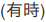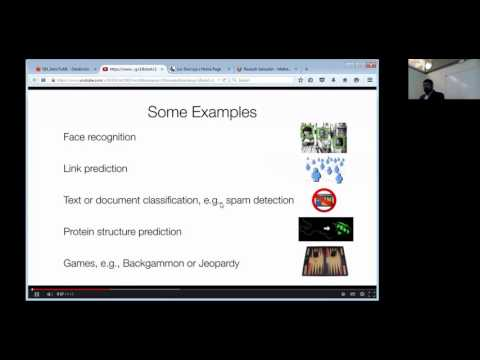// Databricks notebook source exported at Sat, 18 Jun 2016 11:13:05 UTC

# Scalable Data Science

### prepared by Raazesh Sainudiin and Sivanand Sivaram

The html source url of this databricks notebook and its recorded Uji:SOURCE: This is from the databricks Community Edition that has been added to this databricks shard at Workspace -> scalable-data-science -> xtraResources -> dbCE -> MLlib -> supervised -> classificationDecisionTrees -> handWrittenDigitRecognition as extra resources for this project-focussed course Scalable Data Science.

# Decision Trees for handwritten digit recognition

This notebook demonstrates learning a Decision Tree using Spark’s distributed implementation. It gives the reader a better understanding of some critical hyperparameters for the tree learning algorithm, using examples to demonstrate how tuning the hyperparameters can improve accuracy.

Background: To learn more about Decision Trees, check out the resources at the end of this notebook. The visual description of ML and Decision Trees provides nice intuition helpful to understand this notebook, and Wikipedia gives lots of details.

Data: We use the classic MNIST handwritten digit recognition dataset. It is from LeCun et al. (1998) and may be found under “mnist” at the LibSVM dataset page.

Goal: Our goal for our data is to learn how to recognize digits (0 - 9) from images of handwriting. However, we will focus on understanding trees, not on this particular learning problem.

Takeaways: Decision Trees take several hyperparameters which can affect the accuracy of the learned model. There is no one “best” setting for these for all datasets. To get the optimal accuracy, we need to tune these hyperparameters based on our data.

## Let’s Build Intuition for Learning with Decision Trees

``````
//This allows easy embedding of publicly available information into any other notebook
//when viewing in git-book just ignore this block - you may have to manually chase the URL in frameIt("URL").
//Example usage:
// displayHTML(frameIt("https://en.wikipedia.org/wiki/Latent_Dirichlet_allocation#Topics_in_LDA",250))
def frameIt( u:String, h:Int ) : String = {
"""<iframe
src=""""+ u+""""
width="95%" height="""" + h + """"
sandbox>
<p>
<a href="http://spark.apache.org/docs/latest/index.html">
Fallback link for browsers that, unlikely, don't support frames
</a>
</p>
</iframe>"""
}
displayHTML(frameIt("https://en.wikipedia.org/wiki/Decision_tree_learning",500))

``````

## Load MNIST training and test datasets

Our datasets are vectors of pixels representing images of handwritten digits. For example:These datasets are stored in the popular LibSVM dataset format. We will load them using MLlib’s LibSVM dataset reader utility.

``````
import org.apache.spark.mllib.util.MLUtils
// We convert the RDDs to DataFrames to use with ML Pipelines.
val training = trainingRDD.toDF()
val test = testRDD.toDF()

// Cache data for multiple uses.
training.cache()
test.cache()

println(s"We have \${training.count} training images and \${test.count} test images.")

``````

Note: In Spark 1.6 and later versions, Spark SQL has a LibSVM data source. The above cell can be simplified to:

``````val training = sqlContext.read.format("libsvm").load("/mnt/mllib/mnist-digits-csv/mnist-digits-train.txt")
``````

Display our data. Each image has the true label (the `label` column) and a vector of `features` which represent pixel intensities (see below for details of what is in `training`).

``````
display(training)

``````

The pixel intensities are represented in `features` as a sparse vector, for example the first observation, as seen in row 1 of the output to `display(training)` below, has `label` as `5`, i.e. the hand-written image is for the number 5. And this hand-written image is the following sparse vector (just click the triangle to the left of the feature in first row to see the following):

``````type: 0
size: 780
indices: [152,153,155,...,682,683]
values: [3, 18, 18,18,126,...,132,16]
``````

Here

• `type: 0` says we hve a sparse vector.
• `size: 780` says the vector has 780 indices in total
• these indices from 0,…,779 are a unidimensional indexing of the two-dimensional array of pixels in the image
• `indices: [152,153,155,...,682,683]` are the indices from the `[0,1,...,779]` possible indices with non-zero values
• a value is an integer encoding the gray-level at the pixel index
• `values: [3, 18, 18,18,126,...,132,16]` are the actual gray level values, for example:
• at pixed index `152` the gray-level value is `3`,
• at index `153` the gray-level value is `18`,
• …, and finally at
• at index `683` the gray-level value is `18`

## Train a Decision Tree

We begin by training a decision tree using the default settings. Before training, we want to tell the algorithm that the labels are categories 0-9, rather than continuous values. We use the `StringIndexer` class to do this. We tie this feature preprocessing together with the tree algorithm using a `Pipeline`. ML Pipelines are tools Spark provides for piecing together Machine Learning algorithms into workflows. To learn more about Pipelines, check out other ML example notebooks in Databricks and the ML Pipelines user guide. Also See mllib-decision-tree.html#basic-algorithm.

``````
displayHTML(frameIt("http://spark.apache.org/docs/latest/mllib-decision-tree.html#basic-algorithm",500))

``````
``````
displayHTML(frameIt("http://spark.apache.org/docs/latest/ml-guide.html#main-concepts-in-pipelines", 500))

``````
``````
// Import the ML algorithms we will use.
import org.apache.spark.ml.classification.{DecisionTreeClassifier, DecisionTreeClassificationModel}
import org.apache.spark.ml.feature.StringIndexer
import org.apache.spark.ml.Pipeline

``````
``````
// StringIndexer: Read input column "label" (digits) and annotate them as categorical values.
val indexer = new StringIndexer().setInputCol("label").setOutputCol("indexedLabel")
// DecisionTreeClassifier: Learn to predict column "indexedLabel" using the "features" column.
val dtc = new DecisionTreeClassifier().setLabelCol("indexedLabel")
// Chain indexer + dtc together into a single ML Pipeline.
val pipeline = new Pipeline().setStages(Array(indexer, dtc))

``````

Now, let’s fit a model to our data.

``````
val model = pipeline.fit(training)

``````

We can inspect the learned tree by displaying it using Databricks ML visualization. (Visualization is available for several but not all models.)

``````
// The tree is the last stage of the Pipeline.  Display it!
val tree = model.stages.last.asInstanceOf[DecisionTreeClassificationModel]
display(tree)

``````

Above, we can see how the tree makes predictions. When classifying a new example, the tree starts at the “root” node (at the top). Each tree node tests a pixel value and goes either left or right. At the bottom “leaf” nodes, the tree predicts a digit as the image’s label.

## Hyperparameter Tuning

Run the next cell and come back into hyper-parameter tuning for a couple minutes.

``````
displayHTML(frameIt("https://en.wikipedia.org/wiki/Hyperparameter_optimization", 400))

``````

## Exploring “maxDepth”: training trees of different sizes

In this section, we test tuning a single hyperparameter `maxDepth`, which determines how deep (and large) the tree can be. We will train trees at varying depths and see how it affects the accuracy on our held-out test set.

Note: The next cell can take about 1 minute to run since it is training several trees which get deeper and deeper.

``````
val variedMaxDepthModels = (0 until 8).map { maxDepth =>
// For this setting of maxDepth, learn a decision tree.
dtc.setMaxDepth(maxDepth)
// Create a Pipeline with our feature processing stage (indexer) plus the tree algorithm
val pipeline = new Pipeline().setStages(Array(indexer, dtc))
// Run the ML Pipeline to learn a tree.
pipeline.fit(training)
}

``````
``````
// Define an evaluation metric.  In this case, we will use "precision," which is equivalent to 0-1 accuracy.
import org.apache.spark.ml.evaluation.MulticlassClassificationEvaluator
val evaluator = new MulticlassClassificationEvaluator().setLabelCol("indexedLabel").setMetricName("precision")

``````
``````
// For each maxDepth setting, make predictions on the test data, and compute the accuracy metric.
val accuracies = (0 until 8).map { maxDepth =>
val model = variedMaxDepthModels(maxDepth)
// Calling transform() on the test set runs the fitted pipeline.
// The learned model makes predictions on each test example.
val predictions = model.transform(test)
// Calling evaluate() on the predictions DataFrame computes our accuracy metric.
(maxDepth, evaluator.evaluate(predictions))
}.toDF("maxDepth", "accuracy")

``````

We can display our accuracy results and see immediately that deeper, larger trees are more powerful classifiers, achieving higher accuracies.

Note: When you run `display()`, you will get a table. Click on the plot icon below the table to create a plot, and use “Plot Options” to adjust what is displayed.

``````
display(accuracies)

``````

Even though deeper trees are more powerful, they are not always better. If we kept increasing the depth, training would take longer and longer. We also might risk overfitting (fitting the training data so well that our predictions get worse on test data); it is important to tune parameters based on held-out data to prevent overfitting.

## Exploring “maxBins”: discretization for efficient distributed computing

This section explores a more expert-level setting `maxBins`. For efficient distributed training of Decision Trees, Spark and most other libraries discretize (or “bin”) continuous features (such as pixel values) into a finite number of values. This is an important step for the distributed implementation, but it introduces a tradeoff: Larger `maxBins` mean your data will be more accurately represented, but it will also mean more communication (and slower training).

The default value of `maxBins` generally works, but it is interesting to explore on our handwritten digit dataset. Remember our digit image from above:It is grayscale. But if we set `maxBins = 2`, then we are effectively making it a black-and-white image, not grayscale. Will that affect the accuracy of our model? Let’s see!

Note: The next cell can take about 35 seconds to run since it trains several trees. Read the details on `maxBins` at mllib-decision-tree.html#split-candidates.

``````
displayHTML(frameIt("http://spark.apache.org/docs/latest/mllib-decision-tree.html#split-candidates",300))

``````
``````
dtc.setMaxDepth(6) // Set maxDepth to a reasonable value.
val accuracies = Seq(2, 4, 8, 16, 32).map { case maxBins =>
// For this value of maxBins, learn a tree.
dtc.setMaxBins(maxBins)
val pipeline = new Pipeline().setStages(Array(indexer, dtc))
val model = pipeline.fit(training)
// Make predictions on test data, and compute accuracy.
val predictions = model.transform(test)
(maxBins, evaluator.evaluate(predictions))
}.toDF("maxBins", "accuracy")
display(accuracies)

``````

We can see that extreme discretization (black and white) hurts accuracy, but only a bit. Using more bins increases the accuracy (but also makes learning more costly).

#### What’s next?

• Explore: Try out tuning other parameters of trees—or even ensembles like Random Forests or Gradient-Boosted Trees.
• Automated tuning: This type of tuning does not have to be done by hand. (We did it by hand here to show the effects of tuning in detail.) MLlib provides automated tuning functionality via `CrossValidator`. Check out the other Databricks ML Pipeline guides or the Spark ML user guide for details.

Resources

If you are interested in learning more on these topics, these resources can get you started:

Updated: